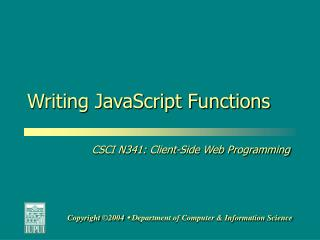DownloadDownload PresentationWriting JavaScript Functions

# Writing JavaScript Functions

Download Presentation## Writing JavaScript Functions

- - - - - - - - - - - - - - - - - - - - - - - - - - - E N D - - - - - - - - - - - - - - - - - - - - - - - - - - -
##### Presentation Transcript

1. Writing JavaScript Functions

2. Goals By the end of this unit, you should understand … • How to breakdown applications into individual, re-usable modules. • How to develop JavaScript functions using a well-defined, well-planned methodology. • How to identify module inputs, processes and outputs.

3. Steps for Writing Functions • Identify the inputs, processes and outputs that your main function will handle. • Identify the inputs, processes and outputs that other functions will handle. • Develop pseudocode for each of your functions. • Code your program.

4. Guidelines for Writing Functions • Have your main function handle (a) getting initial inputs from the user, (b) processing of those inputs (if necessary) and (c) outputs to the user ONLY. Your main function should do little else. • Keep function length short – about 1 editor screen per function, give or take. • Use the main function as a mechanism for communicating among functions.

5. Let’s Try One! • PROBLEM:“Develop an application for car loans. Your application will ask for the sales price, down payment amount, APR and the length of the loan (in months). Using the formula on the next screen, your program will return a monthly payment.”

6. Formula for Monthly Payment

7. Step 1a – Identify Inputs for main() • Yes/No to question “Do you want to calculate the monthly payment for a car loan?” (Validate for Yes/No) • Sales Price (Validate for Number) • Down Payment (Validate for Number) • APR (Validate for Number) • Length of Loan (In Months; Validate for Number)

8. Step 1b – Identify Processes for main() • Call Yes/No validation function • Call number validation function • Calculate Loan Amount:Car Price – Down Payment • Convert APR from percentage format to floating point number:APR / 100 • Call function to calculate monthly payment • Concatenate output message

9. Step 1c – Output for main() • Output a message that includes loan amount, APR, monthly payment and loan length.

10. Step 2 for Yes/No Validator Function • Inputs: • Question to Ask (get from main()) • Default Text (get from main()) • Processes • Ask user the question and see if their answer did not equal “YES” AND did not equal “NO.” • If the user didn’t answer “YES” or “NO”, let them know that “YES” and “NO” are the only acceptable answers. Ask the user the question again until they answer “YES” or “NO.” • Outputs • Give the validated answer back to main().

11. Step 2 for Number Validator Function • Inputs: • Question to Ask (get from main()) • Default Text (get from main()) • Processes • Ask the user to enter a number and check to see if they entered data that can be converted to a number. • If the user didn’t enter numeric data, let them know that the program only accepts numbers. Ask the user the question again until they enter numeric data. • Outputs • Give the validated answer back to main().

12. Step 2 for Calculate Monthly Payment Function • Inputs: • Loan Amount • APR • Length of the Loan, in months • Processes • Figure the monthly interest:APR / 12 • Use the formula below to calculate monthly payment • Outputs • Give the monthly payment back to main().

13. Step 3: Develop Pseudocode • NOTE: Instructor will give you pseudocode in class.

14. Step 4: Code the Program • Finished Code: http://www.cs.iupui.edu/~rmolnar/n341/examples/n341WritingFunctions.html

15. Let’s Try Another One! • PROBLEM:“Develop an application to calculate a student’s semester grade. Your application will ask for the scores from three exams. The application will then calculate the average of the exam scores and return the average along with a letter grade. The teacher uses a standard grading scale.”

16. The Coded Product • Finished Code: http://www.cs.iupui.edu/~rmolnar/n341/examples/n341FunctionsPassingValues.html

17. Commenting Functions • In your code, you need to provide descriptive comments for each of your functions. • When writing function comments, include the following: • A brief (less than 1 line) description of the function • A list of arguments for the function • A list of values returned by the function • A statement of the function’s purpose

18. Commenting Functions • Example: /*FUNCTION: calcAvg() * *PARAMETERS: IntX, IntY, IntZ – All *integer numbers * *RETURNS: The calculated average; *stored in local variable rtnAvg * *PURPOSE: To calculate the average *of three integer values, passed *by a calling procedure and then *return that average to the procedure. */

19. Questions?